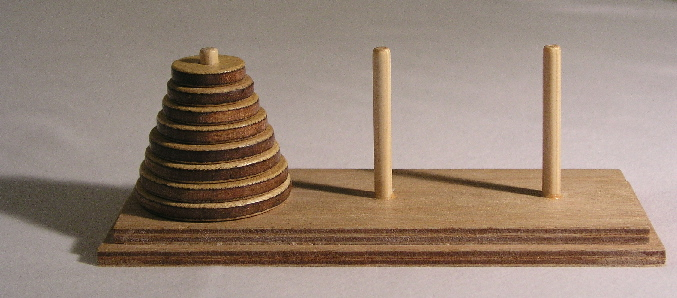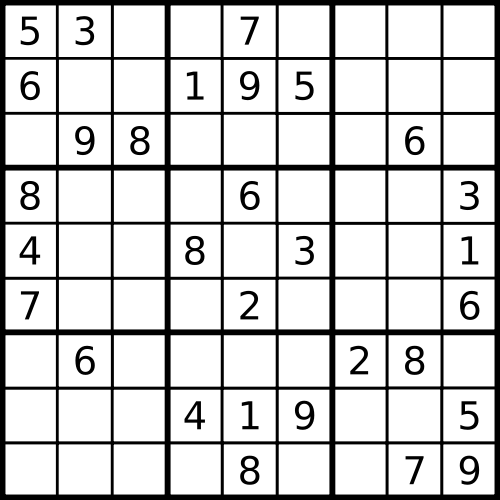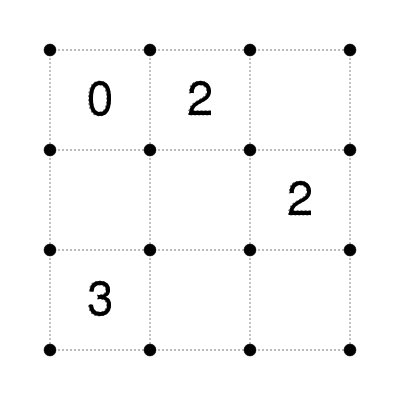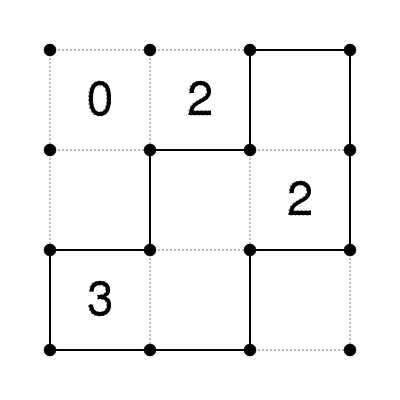# Solving Puzzles with Python

PyCon India 2012
September 28, 2011

# Overview

• Towers of hanoi
• [Learn recursion]
• The eight queens puzzle
• [Compute permutations]
• Finding just one solution
• [Learn generators and generator expressions]
• The sudoku puzzle
• More puzzles

# Towers of Hanoi# Towers of Hanoi

• Move all but the last disk from A to B
• Move the remaining disk from A to C
• Move all the disks on B to C

# Towers of Hanoi

```def move(disks, src, dest, intermediate):
if not disks:
return
move(disks[1:], src, intermediate, dest)
movedisk(disk, src, dest)
move(disks[1:], intermediate, dest, src)

def movedisk(disk, src, dest):
print "move %d from %s to %s" % (disk, src, dest)

if __name__ == "__main__":
move([3, 2, 1], "A", "B", "C")
```

# Recursion: Exponent

```def exponent(x, n):
# ...
pass
```

# Recursion: Exponent

```def exponent(x, n):
if n == 0:
return 1
else:
return x * exponent(x, n-1)
```

# Recursion: Fast Exponent

```def fast_exponent(x, n):
if n == 0:
return 1
elif n % 2 == 0:
return exponent(x*x, n/2)
else:
return x * exponent(x, n-1)
```

# Recursion: Count Change

How to make change of 100 using denominations 50 and 25?

``````50 + 50
50 + 25 + 25
25 + 25 + 25

3 ways
``````

# Recursion: Count Change

How to make change of 100 using denominations 50, 20 and 10?

``````2 * 50 + 0 * 20 + 0 * 20
1 * 50 + 2 * 20 + 1 * 10
1        1        3
1        0        5
0        5        0
0        4        2
0        3        4
0        2        6
0        1        8
0        0        10

10 different ways
``````

# Recursion: Count Change

How to make change of 100 using denominations 50, 25, 10, 5 and 1?

Too tedious to try it manually. We need to write a program!

# Recursion: Count Change

The number of ways to change amount `A` is equal to:

• the number of ways to change amount `A` using all but the largest coin, plus
• the number of ways to change amount `A - D` using all kinds of coins, where `D` is the denomination of the largest kind of coin.

# Recursion: Count Change

```def count_change(amount, coins):
"""Find the number of ways to change amount using the specified
coin denominations.

Assume that the denominations are specified in the descending order.
"""
# ...
```

# Recursion: Count Change

```def count_change(amount, coins):
if amount == 0:
return 1
elif amount < 0:
return 0
elif len(coins) == 0:
return 0
else:
return count_change(amount-coins, coins) + \
count_change(amount, coins[1:])
```

Lets try some examples.

``````>>> count_change(50, [50, 25, 10, 5])
11
>>> count_change(50, [50, 25, 10, 5, 1])
50
>>> count_change(100, [50, 25, 10, 5, 1])
292
>>> count_change(200, [50, 25, 10, 5, 1])
2435
>>> count_change(200, [50, 25, 10, 5, 2, 1])
58030
``````

# Brute-force

• Compute all possible positions
• keep the ones that are valid solutions

# Presenter Notes

## Generators

Generators simplifies creation of iterators.

```def yrange(n):
i = 0
while i < n:
yield i
i += 1
```

Lets try it out.

```>>> y = yrange(3)
>>> y.next()
0
>>> y.next()
1
>>> y.next()
2
>>> y.next()
Traceback (most recent call last):
File "<stdin>", line 1, in <module>
File "<stdin>", line 14, in next
StopIteration

>>> list(yrange(3))
[0, 1, 2]
```

# Presenter Notes

## More Generators

```def integers():
i = 1
while True:
yield i
i = i + 1

def squares():
for i in integers():
yield i * i

def take(n, seq):
"""Returns first n values from the given sequence."""
seq = iter(seq)
result = []
try:
for i in range(n):
result.append(seq.next())
except StopIteration:
pass
return result
```

Lets try it.

```>>> take(5, squares())
[1, 4, 9, 16, 25]
```

# Presenter Notes

## Generator Expressions

Generator expressions take generators to the next level.

See improved versions of `squares` and `take` functions using generator expressions.

```def integers():
i = 1
while True:
yield i
i = i + 1

def squares():
return (i*i for i in integers())

def take(n, seq):
seq = iter(seq)
return list(seq.next() for i in range(n))
```

See it in action.

```>>> take(5, squares())
[1, 4, 9, 16, 25]
```

# Presenter Notes

## Lazy Permutations

```def permutations(xs):
if not xs:
return [[]]
else:
return ([x] + xs1 for x in xs
for xs1 in permutations(remove(x, xs)))
```

Lets try it:

``````>>> p = permutations(range(5))
>>> p.next()
[0, 1, 2, 3, 4]
>>> p.next()
[0, 1, 2, 4, 3]
>>> p.next()
[0, 1, 3, 2, 4]
``````

# SudokuIdeas?

Recursively!

# How to Solve Sudoku (3)

• find empty position
• find all the possible values there
• for each possible value:
• place that value there
• solve the rest of the puzzle

# Sudoku - solver

```def solve(grid):
pos = find_empty_position(grid)
if not pos:
return grid

ri, ci = pos
for n in find_possible_values(grid, pos):
grid[ri][ci] = n
soln = solve(grid)
if soln:
return soln

# failed to find a solution
# put the original value back
grid[ri][ci] = '.'

# sample puzzle are at http://magictour.free.fr/msk_009
```

# Sudoku - Find empty position

```def find_empty_position(grid):
for row in range(9):
for col in range(9):
if grid[row, col] == '.':
return (row, col)
```

# Sudoku - Find possible values

```def find_possible_values(grid, pos):
return set("123456789") - \
set(get_row(grid, pos)) - \
set(get_col(grid, pos)) - \
set(get_block(grid, pos))
```

# Fence Puzzlehttp://anandology.com/stream/2009/12/fence/puzzle.html

# Loop Puzzlehttp://anandology.com/stream/2010/01/loop/

# Sky Scrapershttp://anandology.com/stream/2010/01/skyscrapers/

# Questions?

Slides and code is available at:

http://github.com/anandology/solving-puzzles-with-python